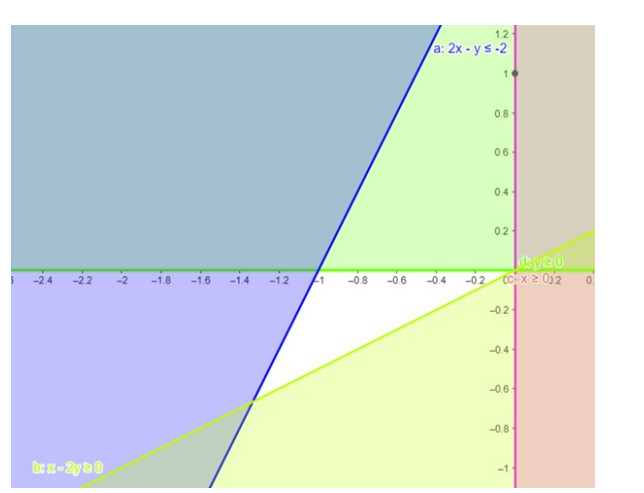# Solve the given inequalities

Question:

Solve the given inequalities $2 x-y \leq-2, x-2 y \geq 0, x \geq 0, y \geq 0$ graphically in two - dimensional plane.

Solution:

The graphical representation of $2 x-y \leq-2, x-2 y \geq 0$

$x \geq 0, y \geq 0$ is given by common region in the figure below.

$2 x-y \leq-2 \ldots \ldots$ (1)

$x-2 y \geq 0 \ldots \ldots$ (2)

$x \geq 0 \ldots \ldots$ (3)

$y \geq 0 \ldots \ldots$ (4)

Inequality (1) represents the region above line $2 x-y=-2$ (including the line $2 x-y=-2$ ).

Inequality (2) represents the region below line $x-2 y=0$ (including the line $x-2 y=0$ ).

Inequality (3) represents the region in front of line $x=0$ (including the line $x=0$ ).

Inequality (4) represents the region above line $y=0$ (including the line $y=0$ ).

Therefore, we can see in the figure that there is no common shaded region.

So there linear inequalities in equations has no solution.

This can be represented as follows,